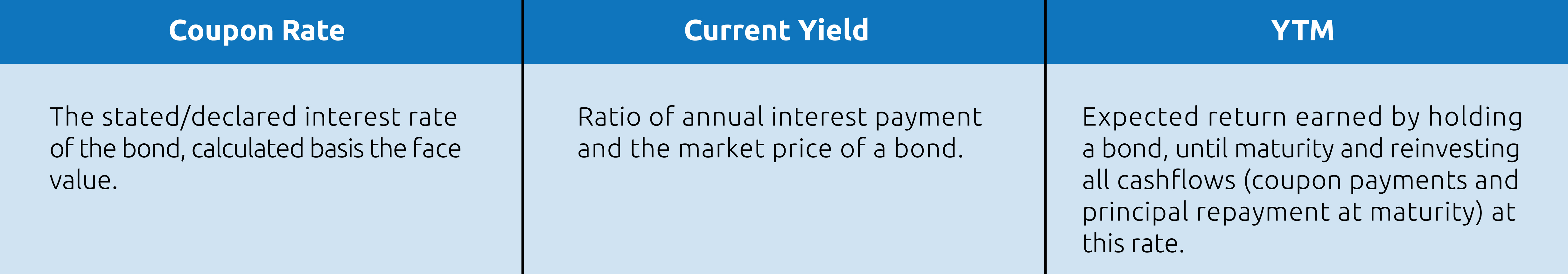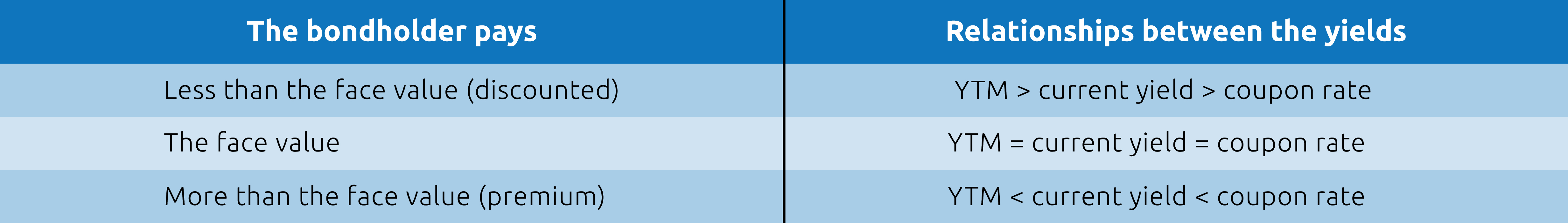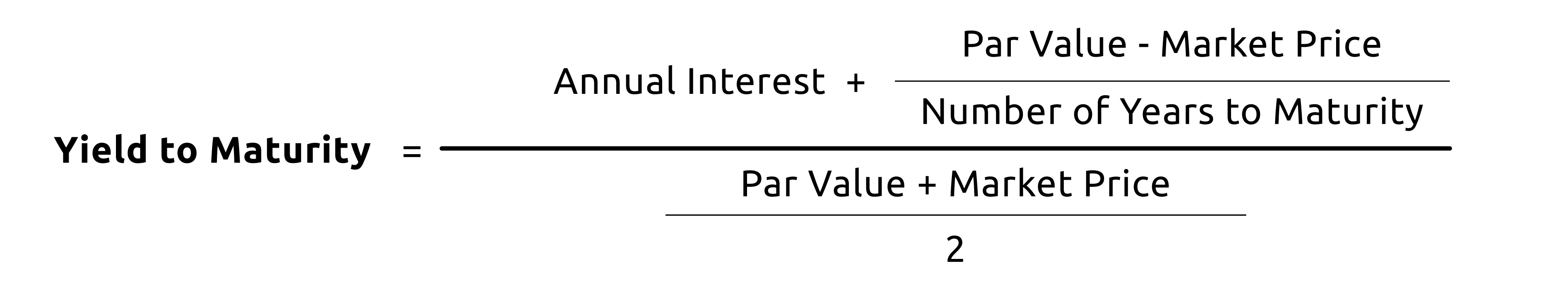# What is Yield to Maturity in Mutual Funds?​​Yield to Maturity (YTM) is the expected return an investor would earn if he/she holds the bond until its maturity. For example, if a bond’s face value is Rs 1000, maturity is 5 years, and coupon is 8%, it implies that if you were to hold the bond for 5 years, then you shall get Rs 80 per year as interest till the 5th year, after which you shall get your principal amount back. At face value, when the bond is first issued, the coupon rate and the yield are the same. While coupon rate, in this case, remains fixed throughout the tenure of the bond. Yields and bond price move in the opposite direction. When bond yields go up, prices go down, and when bond yields go down, prices go up.

## Coupon Rate Vs YTM Vs Current Yield

Before we move further, let us understand that when you purchase a bond, there are three things that are fixed, given below with examples-

1.Face Value- Rs 1000

2.Coupon Rate- 8%

3.Maturity Period- 5 years

Yields can be measured in multiple ways, out of which 3 most common measures are-If there are no changes in the market price of the bonds, interest rates or other external factors that affect a bond’s price, the coupon rate and the current yield will be the same as the YTM. Having said that, the face value at which you bought the bond in the above example is Rs 1000, but the market price of the bond may fluctuate due to rise and fall of the interest rates in the economy, credit risks, demand for the bond, etc.

Taking the same example further, suppose the market price of the bond drops to Rs 950 (discounted rate). With your coupon remaining constant at Rs 80 per year, the current yield becomes= Rs 80/ Rs 950 %= 8.421%.

Similarly, if the market price of the bond becomes Rs 1050 (premium), your current yield will be Rs 80/ Rs 1050 %= 7.619%

Hence, you can see that the current yield is the return at any given time basis the prevailing market price of the bond. When a bond is purchased at face value (Rs 1000 in this case), the current yield is the same as the coupon rate, which in turn is the same as the YTM. But as the market conditions change, the three begin to differ.

Now, if an investor buys this bond at a discounted rate of Rs 950, he will still get Rs 1000 at the time of maturity, thereby increasing his overall yield. This comprehensive yield, which is a relatively better metric to measure the yield, is called YTM.## YTM of a debt mutual fund scheme

YTM minus expense of a debt scheme is the approximate return an investor can expect if ALL the bonds and the other securities in the scheme are held till maturity. It can also be defined as the weighted average of the yields of all the underlying securities in the proportion of the holding. YTM of a debt scheme may not be constant because the debt schemes are actively managed, and the fund manager may change the fund’s portfolio as per the market conditions.

Hence, YTM minus expense can be a rough indicator of how much return an investor can expect if all the securities are held till maturity, but the returns may vary due to interest rates and changes in the portfolio. Reason for your debt scheme showing high YTM can be that the debt mutual fund scheme you are investing in might be taking higher risks. The market price of the bond and its yield are inversely related. If there is a fall in the market price, it pushes the YTM upward

When deciding on a scheme, the investor is advised to look at the YTM in tandem with the underlying portfolio because a high YTM may not mean high return or a good fitment in your portfolio.

Sources-